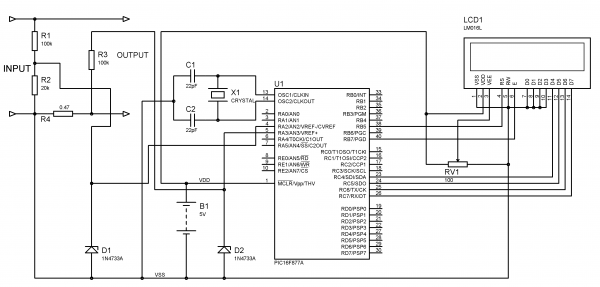# Voltmeter and Ammeter using PIC Microcontroller

Voltmeter and Ammeter can be easily made using PIC Microcontroller having ADC (Analog to Digital Converter). I am using PIC16F877A and the result is displayed on an LCD Display. PIC16F877A is enough if you do this project only for testing purposes. I suggest to use PIC with low pin numbers and multiplexed 7 segment display if you wish to use this as your measuring instrument.If you don’t know the basis of PIC ADC and LCD Interfacing please read the following articles.

ADC module of PIC Microcontroller converts the Signals on its analog pin to 10 bit binary data and it has software selectable high and low voltage reference input to some combination of VDD, VSS, RA2 and RA3. The analog input to PIC is limited to VSS and VDD voltages (0 – 5V) of PIC.

This circuit is designed to measure 0 to 30V. So we will map 0 to 30V to 0 to 5V by using a voltage divider. Current through a circuit can be measured by introducing  a 1 ohm resistor and measuring the voltage across it. To minimize the path resistance we will use .47 ohm special resistor with fuse (shown in figure) and current is calculated. Voltage and Current Sampling circuit is shown below. When the Input voltage is 30V (max) the voltage across 20K ohm resistor becomes 5V which is feedback to the analog pin RA2 of the PIC Microcontroller.

The voltage across .47 ohm resistor is also feedback to the analog pin RA3 via 100K ohm resistor. 5.1V Zener Diode is added in parallel to these analog input pins to protect PIC from over voltages.

The ADC module of PIC converts analog input to 10 bit digital number. We want to convert this digital to corresponding voltage in decimal.

• 0v  =  0 0 0 0
• 5v  =  1  1  1  1

Resolution = (Vref+ – Vref-)/(1024-1)   (as it is 10 bit ADC)

= 5/1023

= 4.887 mV

Thus it means that for a change in 4.887mV, the binary output changes by 1.

So voltage input to the analog pin of PIC can be calculated as follows…

```v = ADC_Read(2);  // ADC value of channel 2 (voltage)
V = v*4.89;       // Converting ADC value to mV
I = i*4.89;       // Converting ADC value to mV```

By using values V and I we can calculate the Input Voltage and Current across the Load (Connected across Output terminals).

Voltage across 20K resistor = V

Current through 20K = V/20K

Input Voltage = Current through 20K * 120K  (Current flowing to PIC can be neglected)

Thus,

`V = (V/20)*120;`

Voltage across 0.47 ohm resistor = V

Current through Load = Current through 0.47 ohm resistor = V/0.47

Thus,

I = I/0.47;

To display the results in LCD Display we need to convert these readings into string, we use the user defined function look() for it. It converts each digit in the reading to corresponding character (see the source code).

# Circuit DiagramFor more detail: Voltmeter and Ammeter using PIC Microcontroller